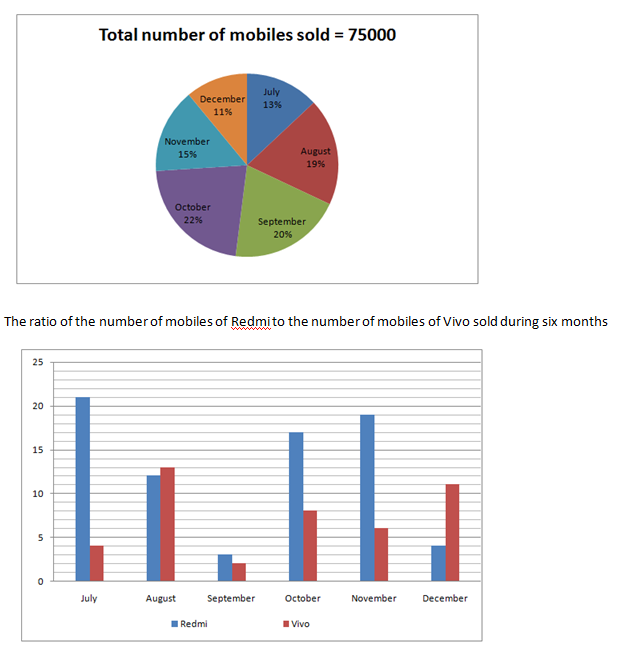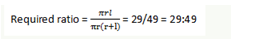# “20-20” Quantitative Aptitude | Crack SBI Clerk 2018 Day-22

Dear Friends, SBI Clerk 2018 Notification has been released we hope you all have started your preparation. Here we have started New Series of Practice Materials specially for SBI Clerk 2018. Aspirants those who are preparing for the exams can use this “20-20” Quantitative Aptitude Questions.

## Daily Practice Test Schedule | Good Luck

 Topic Daily Publishing Time Daily News Papers & Editorials 8.00 AM Current Affairs Quiz 9.00 AM Logical Reasoning 10.00 AM Quantitative Aptitude “20-20” 11.00 AM Vocabulary (Based on The Hindu) 12.00 PM Static GK Quiz 1.00 PM English Language “20-20” 2.00 PM Banking Awareness Quiz 3.00 PM Reasoning Puzzles & Seating 4.00 PM Daily Current Affairs Updates 5.00 PM Data Interpretation / Application Sums (Topic Wise) 6.00 PM Reasoning Ability “20-20” 7.00 PM English Language (New Pattern Questions) 8.00 PM General / Financial Awareness Quiz 9.00 PM

“20-20” Aptitude Questions | Crack SBI Clerk 2018 Day-22

Click “Start Quiz” to attend these Questions and view Explanation

### Click Here for SBI Clerk Prelims and Mains 2018 – Full Length Mock Test

Directions (Q. 1-5): what approximate value will come in place of question mark(?) in the given questions.

1). (36.01)3 × (4096)1/2 × (37.99)2 ÷ (93 × 75.982) = 4?

1. 7
2. 3
3. 5
4. 8
5. 9

2). (4809.01 + 9615.96 + 14425.03) ÷ 4.98+6.02 = (?)2

1. 92
2. 67
3. 72
4. 76
5. 74

3). (35% of 74000) ÷ ? = (123 % of 13.02)2 × 2.01

1. 40
2. 50
3. 75
4. 90
5. 65

4). 4/15 of 393 + 7/12 of 473 = ? × (1.99 + 1.01)

1. 127
2. 137
3. 157
4. 177
5. 147

5). √(2809.001) ÷ 7.98 × (12.01)2+ 46.002 = ?

1. 1300
2. 900
3. 1000
4. 1100
5. 980

Directions (Q. 6-10): What should come in place of question mark (?) in the following number series:

6).   17,   9,   15,   40,   143.5,  ?

1. 505.75
2. 578.5
3. 650.25
4. 578
5. 678.5

7).   3,   5,   13,   49,   241,   ?

1. 1210
2. 1451
3. 1221
4. 1441
5. 1200

8).   508,   256,   130,   67,   35.5,   ?

1. 18.25
2. 19.75
3. 17.25
4. 15.75
5. 17.75

9).    7,   13,   31,   85,   247,   ?

1. 409
2. 727
3. 733
4. 649
5. 444

10).   64,   36,   22,   15,   ?,   9.75

1. 11
2. 12
3. 11.5
4. 11.75
5. 10

Directions (Q. 11-15): Study the following pie-chart and bar graph carefully and answer the questions given below.

Percentage distribution of the number of mobiles sold by a shop during six months

Total number of mobiles sold = 75000

The ratio of the number of mobiles of Redmi to the number of mobiles of Vivo sold during six months1. If 37% of the mobiles of Vivo were sold at a discount in November, how many mobiles of Vivo were sold without any discount during the same month?
1. 1697
2. 1681
3. 1701
4. 1709
5. 1721
1. What is the total number of mobiles of Vivo sold during November and December?
1. 7880
2. 9180
3. 8350
4. 9750
5. 8750
1. The number of mobiles of Redmi sold during July is approximately what per cent of the number of mobiles of Redmi sold during November?
1. 93%
2. 100%
3. 88%
4. 96%
5. 90%
1. What is the ratio of the number of mobiles of Vivo sold during July to that sold during October?
1. 13:44
2. 132:139
3. 75:132
4. 122:39
5. 132 : 39
1. If the dealer earned a profit of 517 on each mobile of Vivo sold during October, what was his total profit earned on the mobile of that company during the same month?
1. 4756740
2. 2729760
3. 2595760
4. 3078790
5. 2098710
1. Simple interest on a certain sum of money for 3 years at 8% per annum is half the compound interest on Rs. 4000 for 2 years at 10% per annum. The sum placed on simple interest is:
1. Rs. 1550
2. Rs. 1650
3. Rs. 1750
4. Rs. 2000
5. Rs. 1450
1. The average marks scored by 12 students is 73. If the scores of Ajay, Akhil and Alok are included, the average becomes 73.6. If Ajay scored 68 marks and Alok scored 6 more marks than Akhil find the marks scored by Akhil.
1. 79
2. 77
3. 75
4. 76
5. 74
1. Three years ago father’s age was 7 times his son’s age. Three years hence the father’s age would be four times that of his son. What are the present ages of father and the son?
1. 42 years, 9 years
2. 36 years, 12 years
3. 45 years, 9 years
4. 35 years, 8 years
5. 48 years, 14 years
1. 2 pipes A and B can fill a tank in 24 minutes and 32 minutes respectively while working alone. If both the pipes are opened simultaneously then after how much time pipe B should be closed so that tank is full in 18 minutes?
1. 5 minutes
2. 12 minutes
3. 6 minutes
4. 10 minutes
5. 8 minutes
1. Calculate the ratio of curved surface area and total surface area of a cone whose diameter is 40m and height is 21m.
1. 21:32
2. 29:49
3. 25:52
4. 18:35
5. None of these

Directions (Q. 1-5):

(36.01)3 × (4096)1/2 × (37.99)2 ÷ (93 × 75.982) = 4?

Or, 4? = [ 363 x √4096 x 382] / 93 × 762

or, (43 x 93 x 43 x 38 x 38) / (93 x 76 × 76)

= (43×43) / (2×2)

Or, 4? = 43 × 42 = 45

? = 5

(4809.01 + 9615.96 + 14425.03) ÷ 4.98 + 6.02 = (?)2

Or, (?)^2 = [(4809 + 9616 + 14425) / 5] +6

= (28850/5) + 6 = 5770 + 6

Or, ?2 = 5776

? = √5776 = 76

(35% of 74000) ÷ ? = (123% of 13.02)2 x 2.01

Or, (35×74000)/ 100 ÷ ? =  [(123×13) / 100]2 x 2

or, 25900 / ? = (15.99)2 × 2

or, (25900/?) = 16×16×2

?= 25900 / (16×16×2) = 50.58 = 50

4/15 of 393 + 7/12 of 473

= ? x (1.99 + 1.01)

or, ? x 3 = (4/15) x 393 + (7/12) x 480

or, ? x 3 = (4/15) x390 + (7/12) x 480

or, ? x 3 = 104 + 280

or, ? = 384/3 = 128 = 127

√2809.001 ÷ 7.98 x (12.01)2+ 46.002 = ?

or, ? = √2809 ÷ 8 x (12)2 + 46

or, ? = (53/8) x (12)2 + 46

or, ? = 954 + 46

? = 1000

Directions (Q. 6-10):

The series is ×0.5 + 0.5, ×1.5 + 1.5, ×2.5 + 2.5, ×3.5 + 3.5, ×4.5 + 4.5

The series is ×2-1, ×3-2, ×4-3, ×5-4, ×6-5

The whole series is /2 + 2

The series is +3 × 2, +3 × 6, +3 × 18, +3 × 54, +3 × 162

The whole series is ×0.5 + 4

Directions (Q. 11-15):

Number of mobiles sold by Vivo in the month of November

= 75000 × 15/100 × 6/25 = 2700

37% of mobiles sold by Vivo at a discount

= 2700 × 37/100

= 999

Number of mobiles sold without discount = 2700 – 999

= 1701

Total number of mobiles of Vivo sold during the month of November and December together

= 75000 × 15/100 × 6/25 + 75000 × 11/100 × 11/15

= 2700 + 6050

= 8750

Total number of mobiles Redmi sold during the month of July

= 75000 × 21/25 × 13/100

= 750 × 21/25 × 13

= 8190

Total number of mobiles of Redmi sold during the month of November

= 75000 × 19/25 × 15/100

= 750 × 19/25 × 15

= 8550

Required % = 8190/8550 × 100

= 95.78

= 96%

Required ratio

= 750 × 13 × 4/25 : 750 × 22 × 8/25

= 13 : 44

Number of mobiles sold in the month of October

= 75000 × 22/100

= 750 × 22 = 16500

Total number of mobiles of Vivo sold/during the month of October

= 16500 × 8/25

= 660 × 8 = 5280

Total profit = 5280 × 517

= 2729760

Let ‘A’ be the amount obtained when Rs. 4000 is compounded annually.

Therefore, A = 4000*(1 + (10/100))2

= 4840

C.I. = Rs. (4840 – 4000) = Rs. 840

S.I. = 840/2 = Rs. 420.

Let ‘P’ be the sum of money invested on simple interest.

Therefore, P = (42*100)/(3*8) = 1750

Total marks of 15 students = 73.6 x 15 = 1104

Total marks of 12 students = 73 x 12 = 876

Total marks of Ajay, Akhil and Alok = 1104 – 876 = 228

Suppose Akhil scored A marks, then Alok scored A+6

68 +A+A+ 6 =228

=>A= 77

Let the present age of father and son be m and n years respectively.

According to the question; (m – 3) = 7(n – 3)

=> m – 3 = 7n – 21

=> 7n – m = 18… (1)

Similarly; (m + 3) = 4(n + 3)

=> m + 3 = 4n + 12

=> m – 4n = 9… (2)

From Equation (1) and Equation (2);

=> 3n = 27

=> n = 9

Also we will get m = 45

Let’s assume pipe B was open for “t” minutes.

Hence according to the question:

18/24+t/32=1

=> t/32=1-3/4

=> t/32=1/4

=> t = 8 min